# Civil Engineering - Hydraulics

### Exercise :: Hydraulics - Section 1

41.

The horizontal component of the force on a curved surface is equal to

 A. weight of liquid vertically below the curved surface B. force on a vetical projection of the curved surface C. product of pressure at its centroid and the area D. weight of liquid retained by the curved area.

Answer: Option B

Explanation:

No answer description available for this question. Let us discuss.

42.

The radius of gyration of the water line of a floating ship is 4 m and its metacentric height is 72.5 cm. The period of oscillation of the ship, is

 A. π B. 2π C. 3π D. 4π E. π/2

Answer: Option C

Explanation:

No answer description available for this question. Let us discuss.

43.

A pipe consisting of several pipes of varying diameters and lengths, may be replaced by an equivalent pipe of diameter D of length

 A.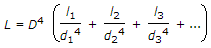B.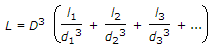C.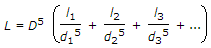D.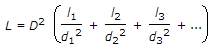Answer: Option C

Explanation:

No answer description available for this question. Let us discuss.

44.

The Empirical formula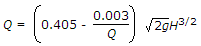for discharge over large rectangular weirs, is known as

 A. Francis formula B. Bazin formula C. Rehbook formula D. Kutter's formula.

Answer: Option B

Explanation:

No answer description available for this question. Let us discuss.

45.

The diameter (d) of a nozzle fixed at the end of a pipe (diameter D, length L) for maximum energy, is

 A.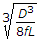B.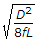C.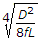D.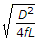Answer: Option C

Explanation:

No answer description available for this question. Let us discuss.

#### Current Affairs 2021

Interview Questions and Answers